# Electrostatics Static Electricity Stationary electricity study of charges

• Slides: 41Electrostatics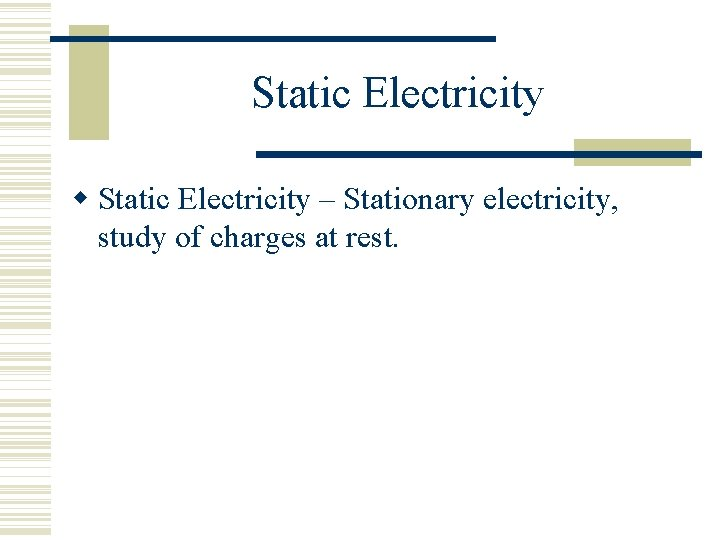Static Electricity – Stationary electricity, study of charges at rest.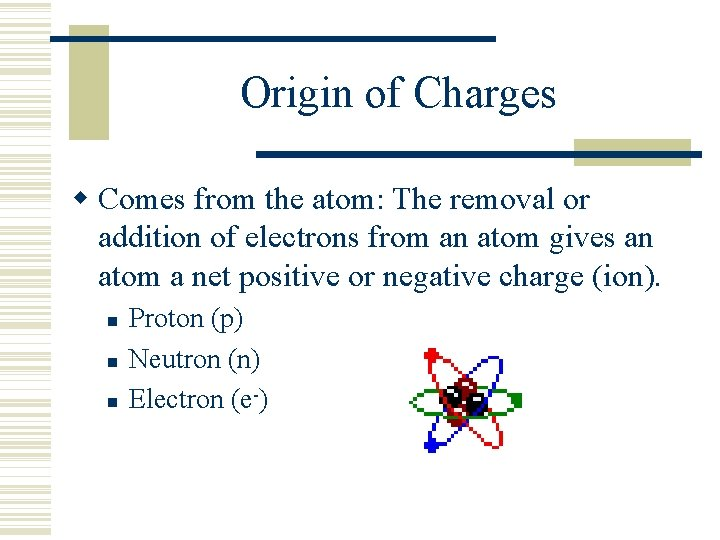Origin of Charges Comes from the atom: The removal or addition of electrons from an atom gives an atom a net positive or negative charge (ion). Proton (p) Neutron (n) Electron (e-)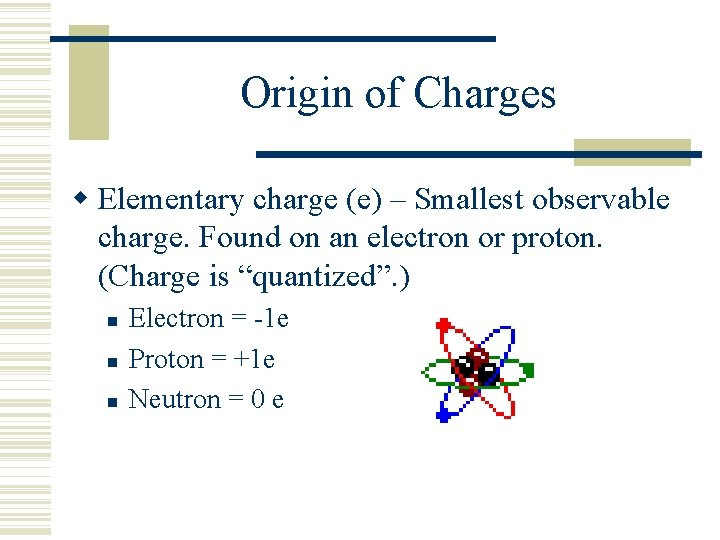Origin of Charges Elementary charge (e) – Smallest observable charge. Found on an electron or proton. (Charge is “quantized”. ) Electron = -1 e Proton = +1 e Neutron = 0 e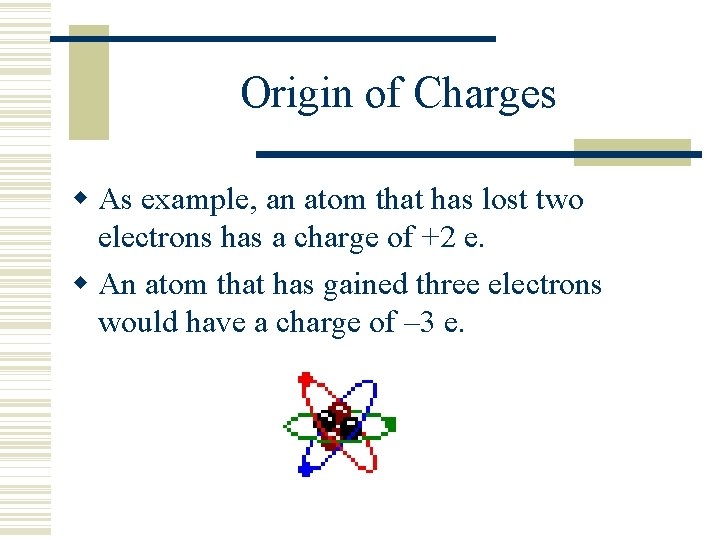Origin of Charges As example, an atom that has lost two electrons has a charge of +2 e. An atom that has gained three electrons would have a charge of – 3 e.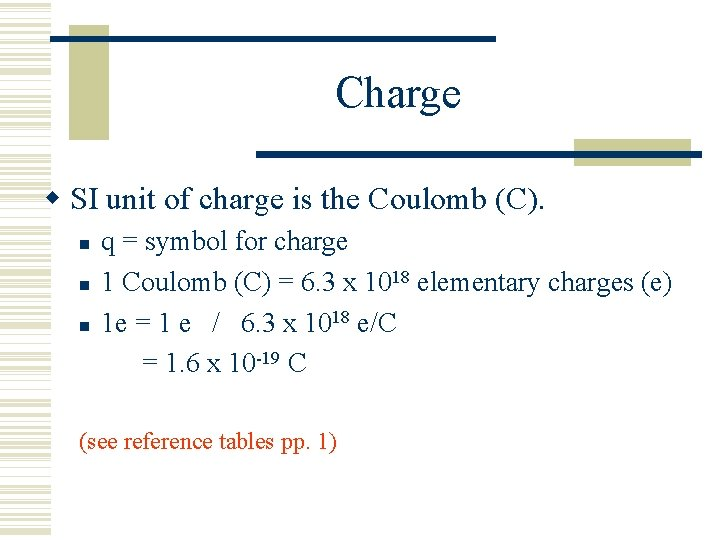Charge SI unit of charge is the Coulomb (C). q = symbol for charge 1 Coulomb (C) = 6. 3 x 1018 elementary charges (e) 1 e = 1 e / 6. 3 x 1018 e/C = 1. 6 x 10 -19 C (see reference tables pp. 1)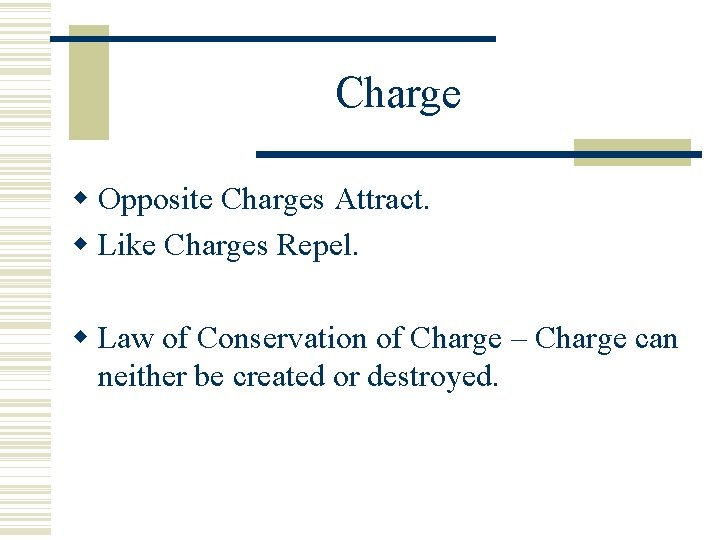Charge Opposite Charges Attract. Like Charges Repel. Law of Conservation of Charge – Charge can neither be created or destroyed.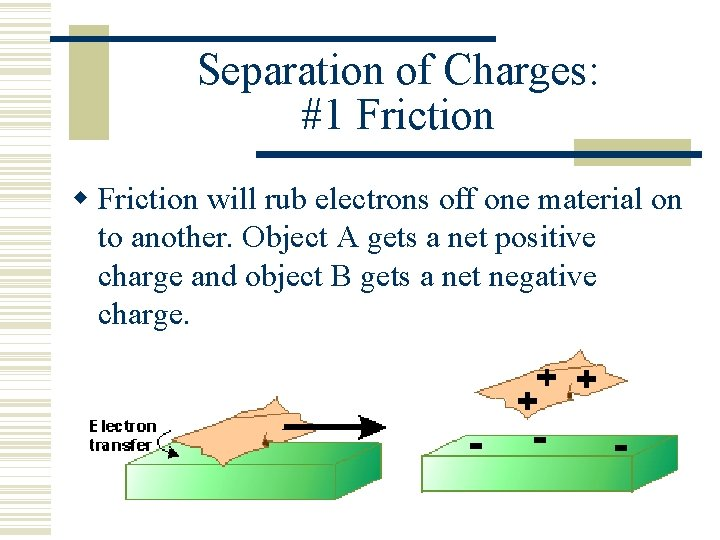Separation of Charges: #1 Friction will rub electrons off one material on to another. Object A gets a net positive charge and object B gets a net negative charge.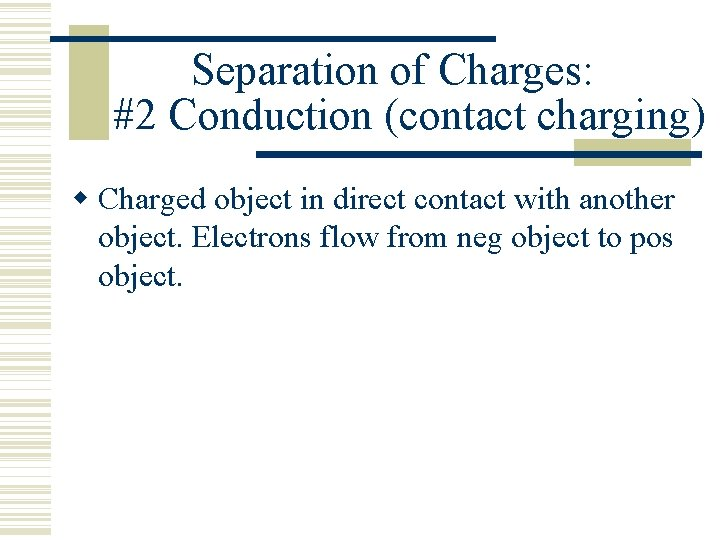Separation of Charges: #2 Conduction (contact charging) Charged object in direct contact with another object. Electrons flow from neg object to pos object.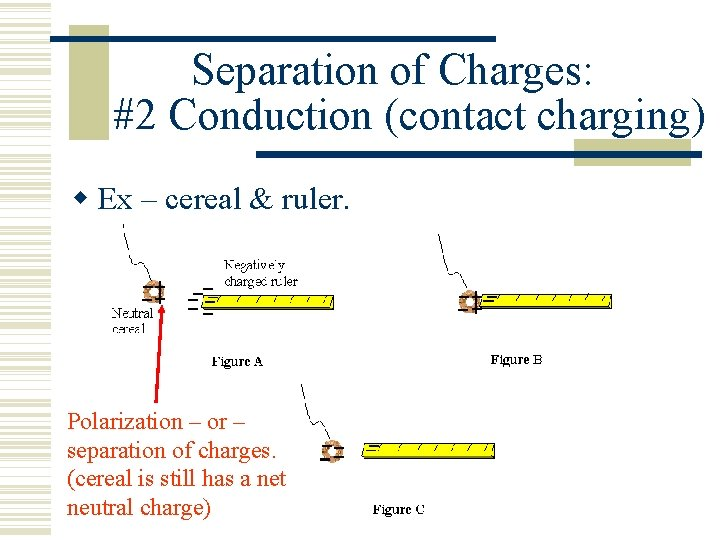Separation of Charges: #2 Conduction (contact charging) Ex – cereal & ruler. Polarization – or – separation of charges. (cereal is still has a net neutral charge)Separation of Charges: #2 Conduction (contact charging) Pith BallsSeparation of Charges: #2 Conduction (contact charging) Ex - Electroscope polarizes in step II. Note: only negative charges (e-) move. + signs mean an ABSENSE of electrons, or a net pos charge.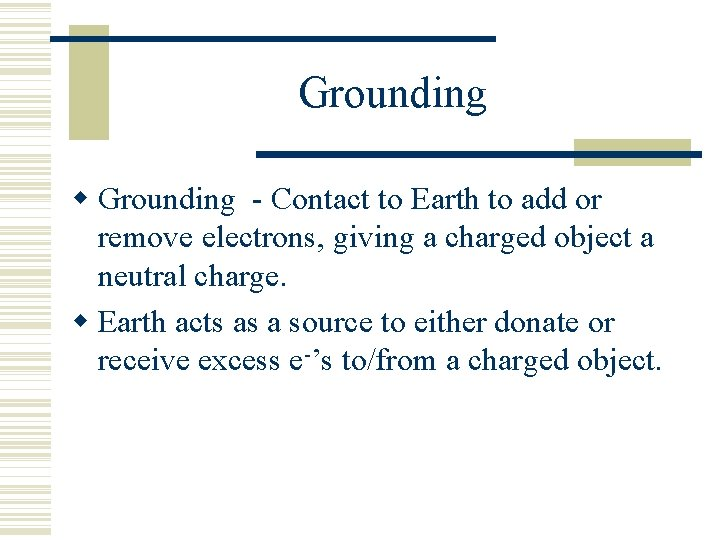Grounding - Contact to Earth to add or remove electrons, giving a charged object a neutral charge. Earth acts as a source to either donate or receive excess e-’s to/from a charged object.Grounding - Contact to Earth to add or remove electrons. Ex – Negatively charged electroscope.Grounding Ex – Positively charged electroscope.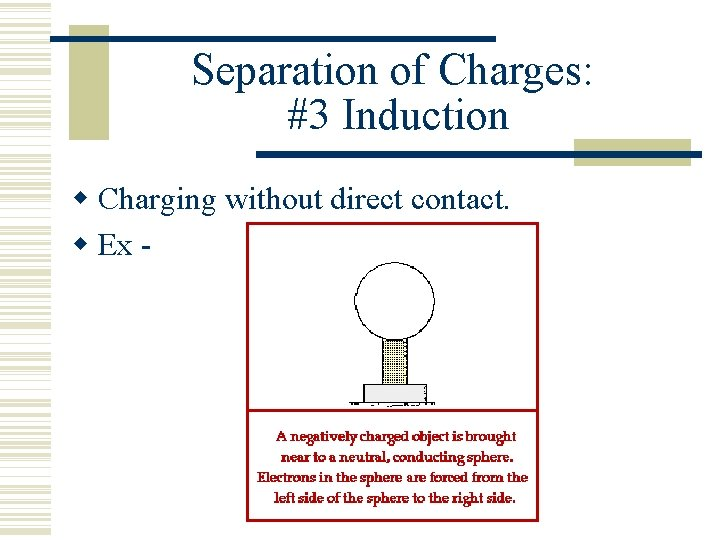Separation of Charges: #3 Induction Charging without direct contact. Ex -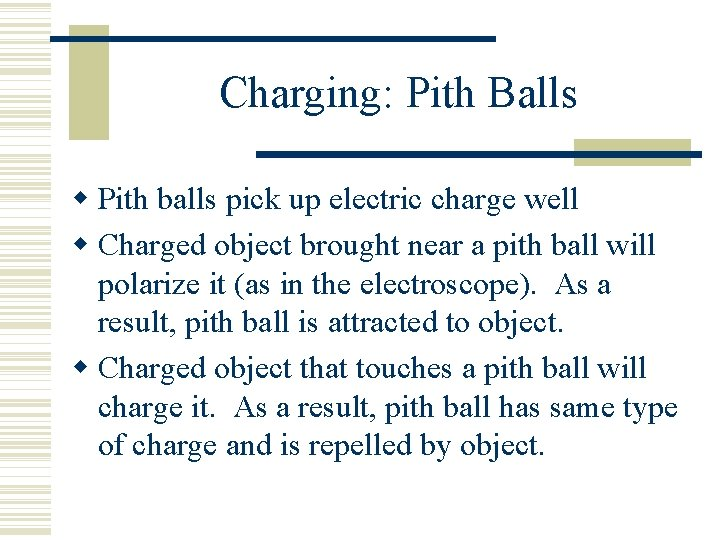Charging: Pith Balls Pith balls pick up electric charge well Charged object brought near a pith ball will polarize it (as in the electroscope). As a result, pith ball is attracted to object. Charged object that touches a pith ball will charge it. As a result, pith ball has same type of charge and is repelled by object.Pith Balls 1. Charged object brought near neutral pith ball. 2. Charged object touches pith ball. 3. Charged object near charged pith ball.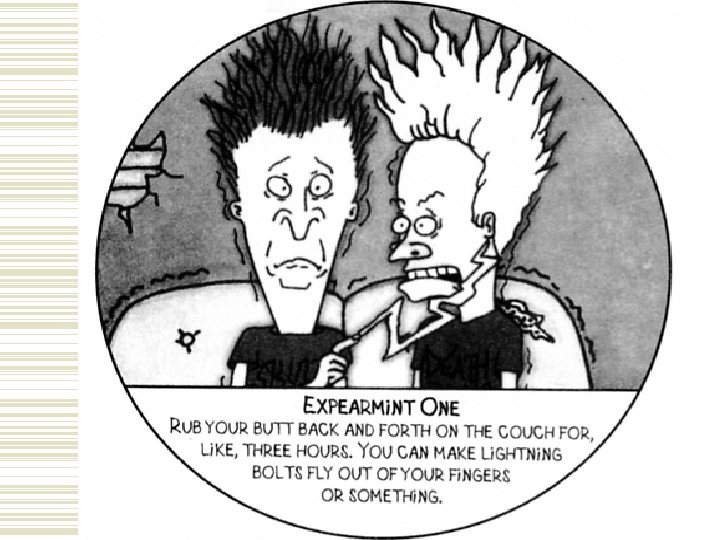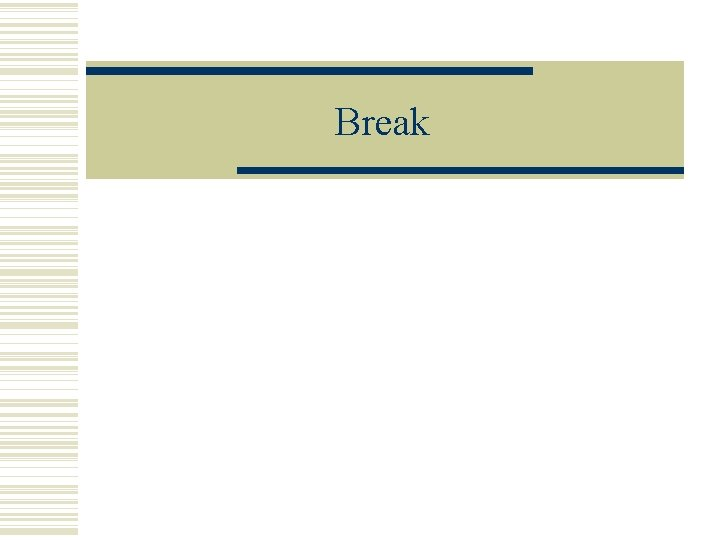Break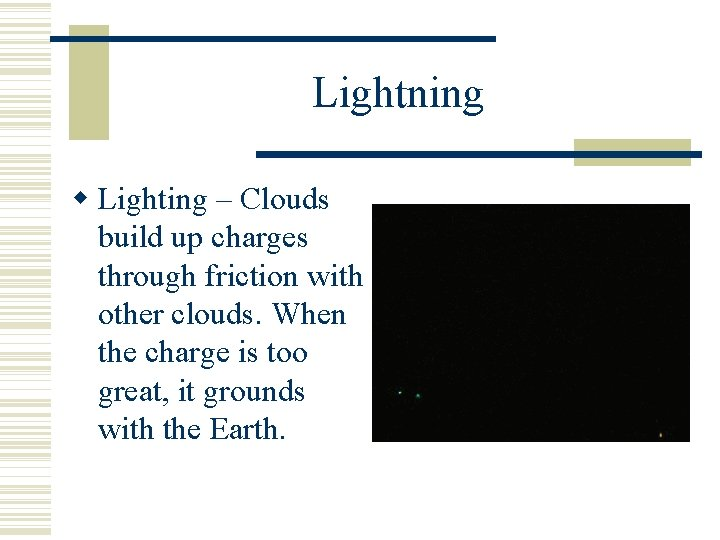Lightning Lighting – Clouds build up charges through friction with other clouds. When the charge is too great, it grounds with the Earth.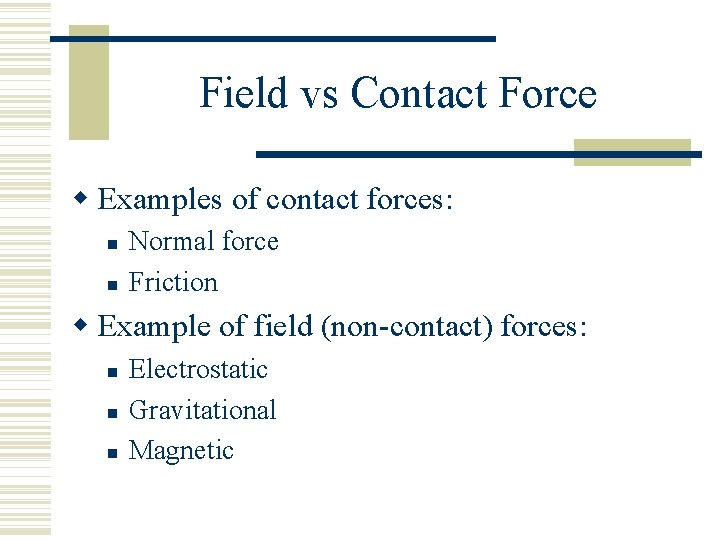Field vs Contact Force Examples of contact forces: Normal force Friction Example of field (non-contact) forces: Electrostatic Gravitational MagneticForces Between Charges Coulomb’s Law – F = electrostatic force k = electrostatic constant r = distance between charges (see reference tables pp 1 & pp 3 “Electricity & Magnetism”)Example #1 What is the force between one proton and one electron where r = 0. 001 m? If F = pos (+) number, the force is repulsive. If F = neg (-) number, the force is attractive.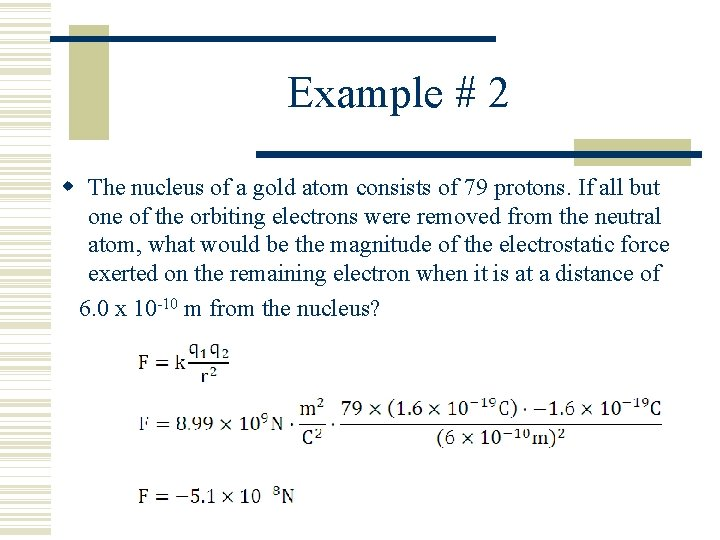Example # 2 The nucleus of a gold atom consists of 79 protons. If all but one of the orbiting electrons were removed from the neutral atom, what would be the magnitude of the electrostatic force exerted on the remaining electron when it is at a distance of 6. 0 x 10 -10 m from the nucleus?BreakElectric Field The region where an electric force acts on a charge. The direction of the field lines point to the direction of which the force is directed on a small positive test charge. (qo = test charge)Electric Field An electric field is said to exist anywhere a force is felt on a positive test charge. Placing a positive test charge in a field and observing its path creates a segment of a "field map. " The movie below demonstrates the tracing of the path of a positive test charge.Electric Field When you have traced the paths of that test charge many times in many different places, you start to get an idea of what the field map looks like. The completed field map is shown below.Electric Field It is very important that you notice that the map lines have a direction to them. The direction represents the motion of the positive test charge when placed at different points around the field. You should also notice that field lines never cross each other. Even as we create other field maps, we find the field lines never cross. The closer the field lines are to each other, the greater the field intensity or field strength.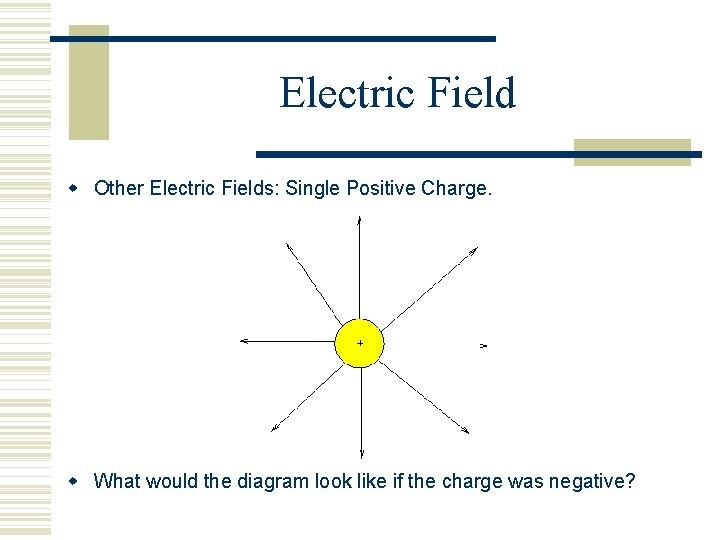Electric Field Other Electric Fields: Single Positive Charge. What would the diagram look like if the charge was negative?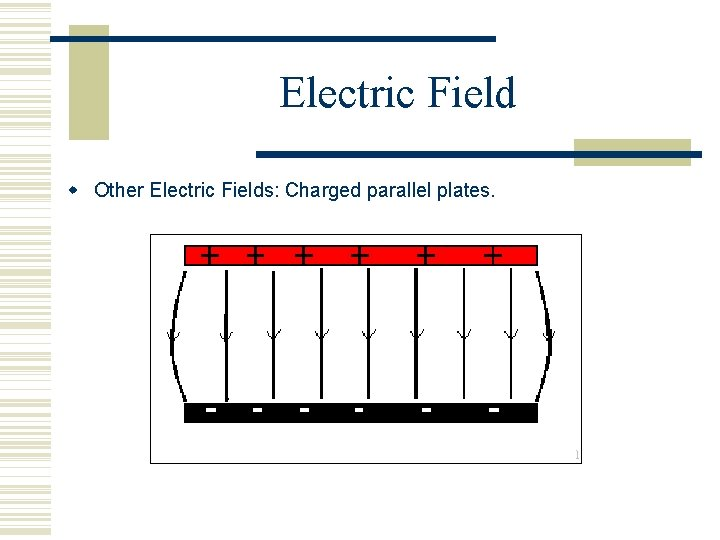Electric Field Other Electric Fields: Charged parallel plates.Electric Field Intensity (Field Strength) – Shows the strength of the electric field, or the force per unit charge at a distance away from a charged object.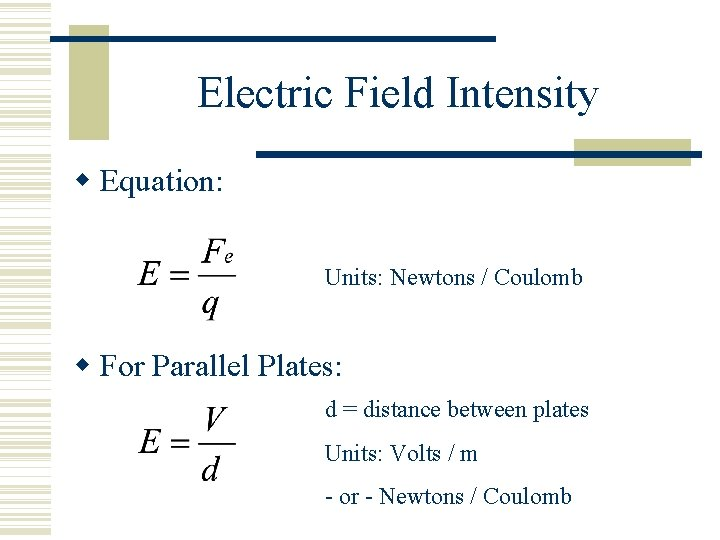Electric Field Intensity Equation: Units: Newtons / Coulomb For Parallel Plates: d = distance between plates Units: Volts / m - or - Newtons / Coulomb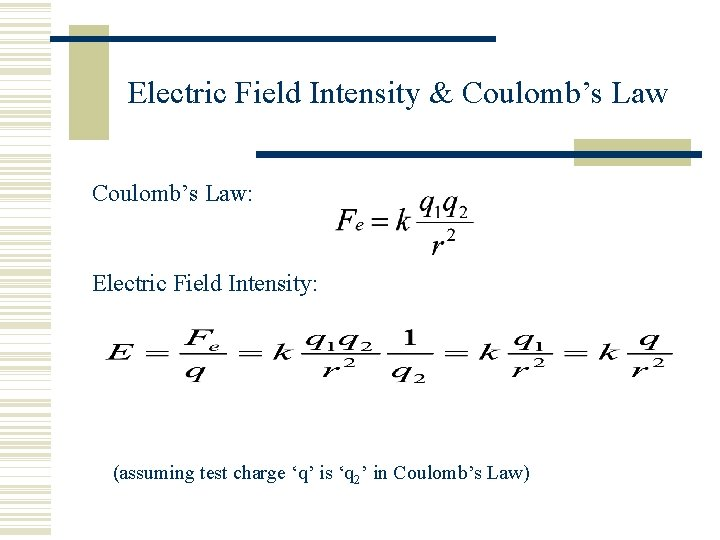Electric Field Intensity & Coulomb’s Law: Electric Field Intensity: (assuming test charge ‘q’ is ‘q 2’ in Coulomb’s Law)Voltage Electric Potential (Voltage)(Potential Difference) – Change in PE per unit of charge. Units: Joules / C - or – Volts (V)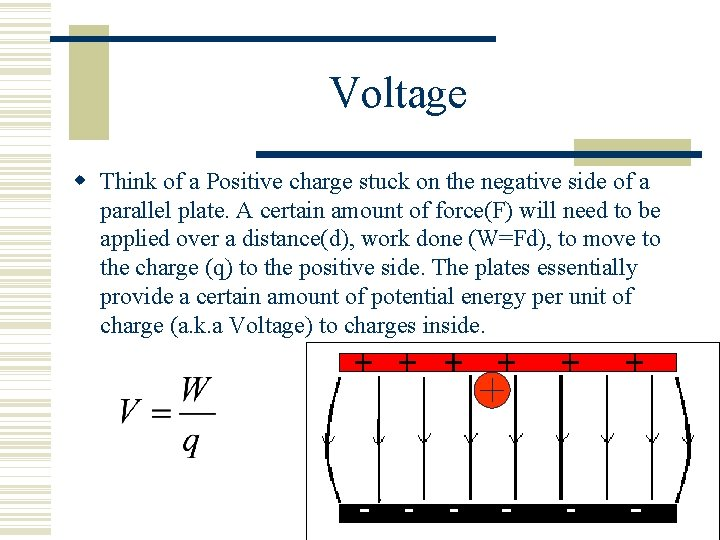Voltage Think of a Positive charge stuck on the negative side of a parallel plate. A certain amount of force(F) will need to be applied over a distance(d), work done (W=Fd), to move to the charge (q) to the positive side. The plates essentially provide a certain amount of potential energy per unit of charge (a. k. a Voltage) to charges inside.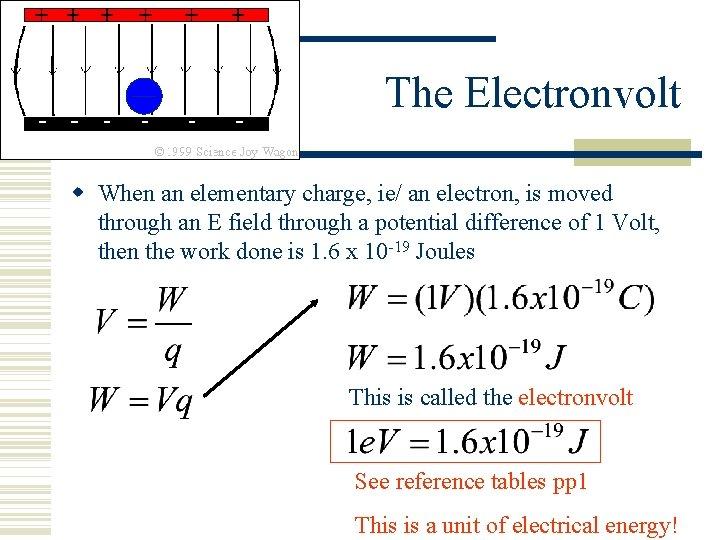The Electronvolt When an elementary charge, ie/ an electron, is moved through an E field through a potential difference of 1 Volt, then the work done is 1. 6 x 10 -19 Joules This is called the electronvolt See reference tables pp 1 This is a unit of electrical energy!How DO You Measure Electric Charge? Millikan oil drop experiment: What forces are acting on the oil drops that are in the uniform electric field?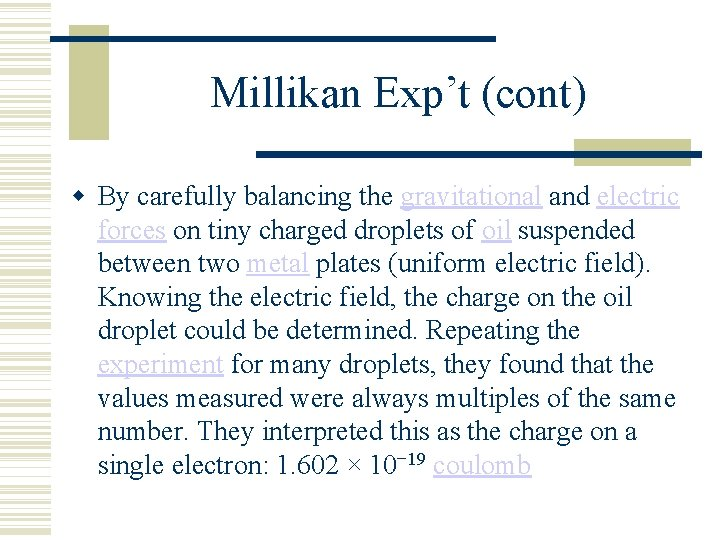Millikan Exp’t (cont) By carefully balancing the gravitational and electric forces on tiny charged droplets of oil suspended between two metal plates (uniform electric field). Knowing the electric field, the charge on the oil droplet could be determined. Repeating the experiment for many droplets, they found that the values measured were always multiples of the same number. They interpreted this as the charge on a single electron: 1. 602 × 10− 19 coulombBreak